# 0.展示PTA总分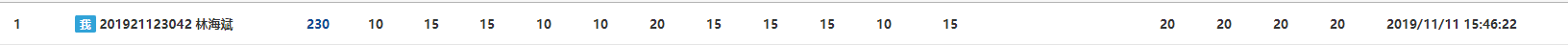# 1.本章学习总结

## 1.1 学习内容总结

• 指针做循环变量做法
设定一个新的指针q指向要循环的指针p
char * q=p;
for(;*q&&*q!='\n';q++)
{

}

• 字符指针如何表示字符串
1：设定一个字符指针p指向一个字符数组的首地址如：
char str={"abcdefg"};
char *p=str;


2:在定义指针的时候将其初始化为字符串char *p={"abcdefg"};

• 动态内存分配
使用malloc函数和calloc函数申请动态内存,之后使用free函数释放掉申请得来的空间如：
char *p=NULL;
p=(char*)malloc(N * sizeof(char));
free(p);

• 指针数组及其应用
指针数组用于存放多个同一类型数据的地址，格式为：类型名 *数组名[数组长度]。如：
char *p;
char q={"abcdefghji"}
for (i = 0; i < 10; i++)
{
p[i]=&q[i];
}

• 二级指针、行指针
指向指针的指针称为二级指针，格式为：类型名 **变量名
行指针为指向数组的指针如：
int a;
int *p;
p=a;


• 函数返回值为指针
若函数的返回值为一个地址，则在定义函数的时候要将函数定义为指针，且返回的指针所指代的地址不能是函数中局部变量中的地址，不然在函数结束时这些数据会被系统收回，使返回的地址错误。

# 2.PTA实验作业

## 2.1合并两个有序数组

### 2.1.1 伪代码

定义void类型的merge函数（形参为int型指针a,int型m,int型指针b,int型n）

i,j,k初始化为0
for 循环条件为k<m+n do
if i==m
for 循环条件为k<m+n do
*(p+k)=b中的元素
b移向下一个元素
if b==n
for 循环条件为k<m+n do
*(p+k)=a中的元素
a移向下一个元素
if a中元素大于b中元素
*(p+k)=b中的元素
b移向下一个元素
else
*(p+k)=a中的元素
a移向下一个元素
for 循环条件为k<m+n do

t移向下一个元素


### 2.1.2 代码截图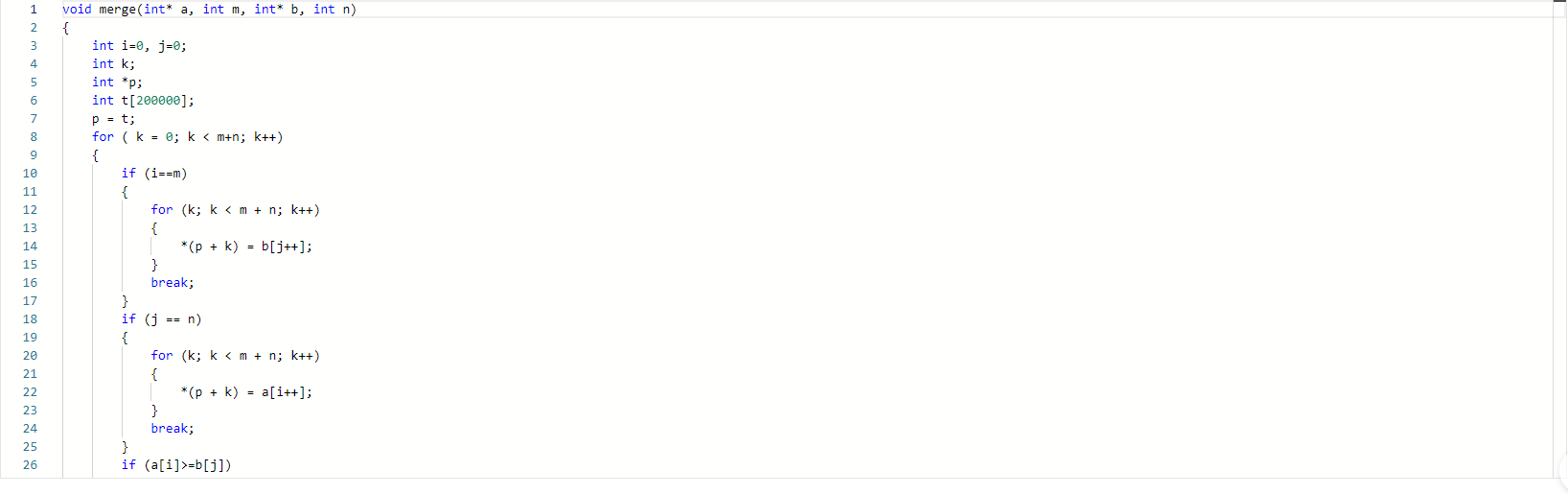• 掌握对数组的排序。
• 掌握两个指针的合并。

### 2.1.4PTA提交列表及说明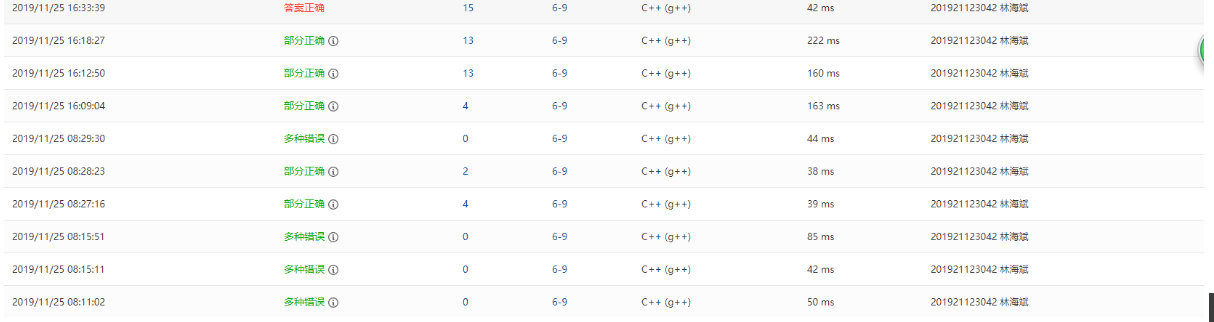## 2.2 字符串的冒泡排序

### 2.2.1伪代码

定义int型变量i,j作为循环变量

for i=0 循环条件为i<N do

for i=0 循环条件为i<K do
for j=0 循环条件为j<N-i-1 do
if s[j]中字符串大于s[j+1]中的字符串

for i=0 循环条件为i<N do



### 2.2.2代码截图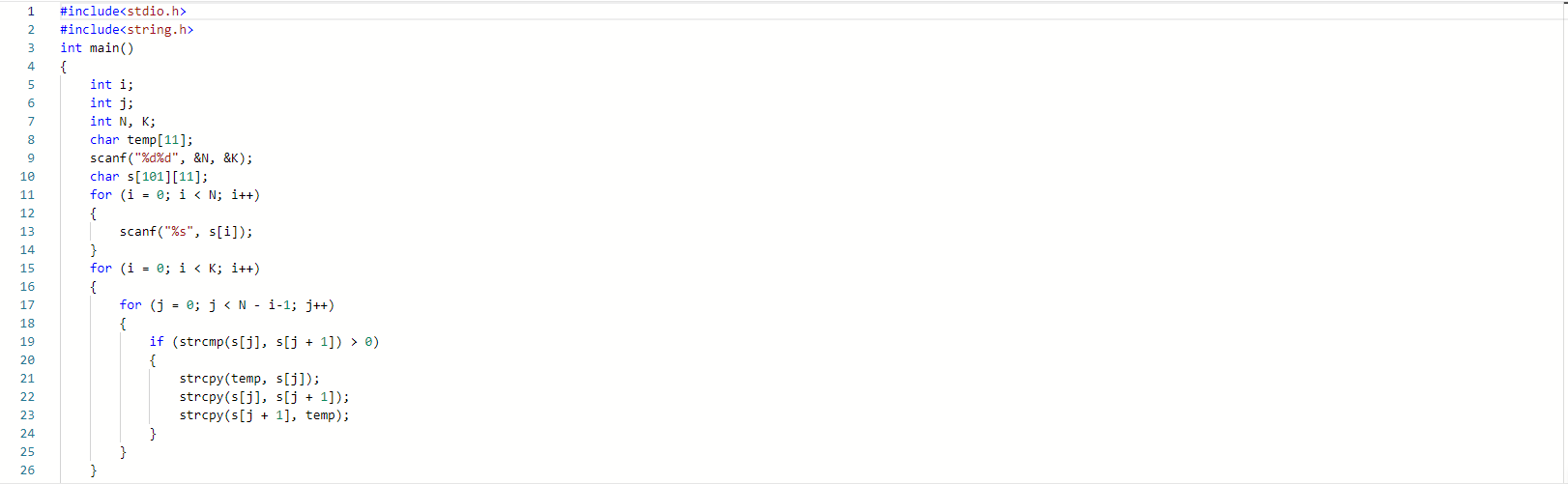### 2.2.3总结本题的知识点

• strcpy语句与strcmp语句的使用
• 冒泡排序的写法，如：
for (i = 0; i < N; i++)
{
for (j = 0; j < N - i-1; j++)
{
if (a[j]>a[j+1])
{
temp=a[j];
a[j]=a[j+1];
a[j+1]=temp;
}
}
}


### 2.2.4PTA提交列表及说明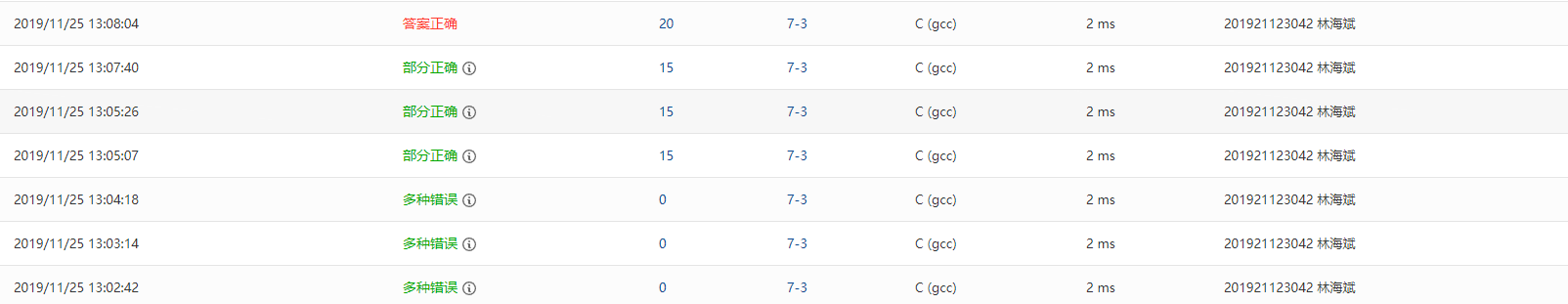## 2.3删除字符串中的子串

### 2.3.1伪代码

定义char型数组mom,s作为题目输入的字符串与字串

while *pta记录字串的首地址为不为空



### 2.3.2代码截图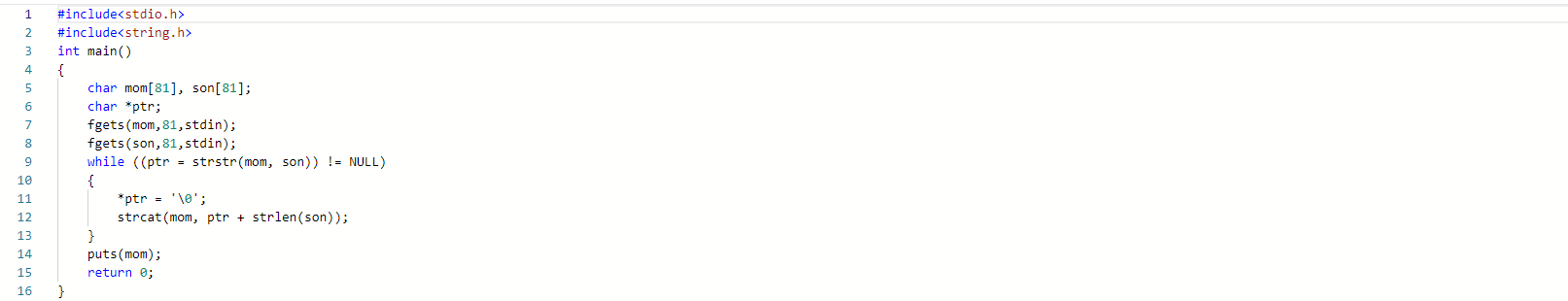### 2.3.3总结本题的知识点

• strstr与strcpy语句的使用，了解到当将一个字符串中间的某个值改为'\0'时，strcpy语句会从'\0'这个位置开始连接要连接的字符串

### 2.3.4PTA提交列表及说明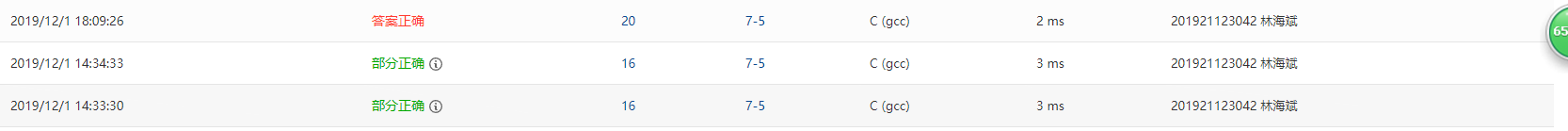# 3.阅读代码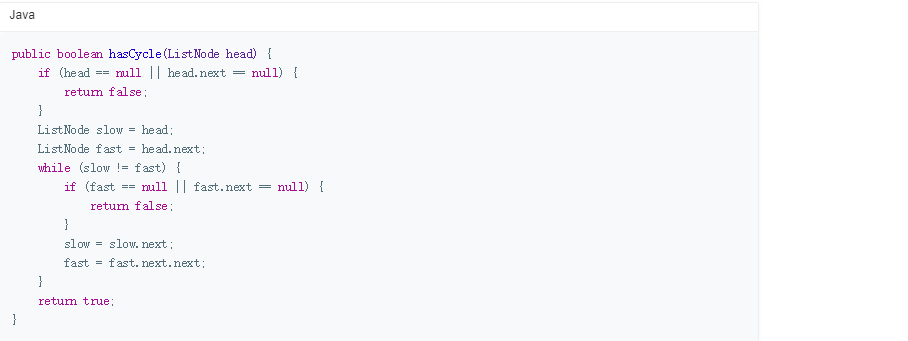posted @ 2019-12-01 14:07  临海文卫  阅读(171)  评论(0编辑  收藏  举报